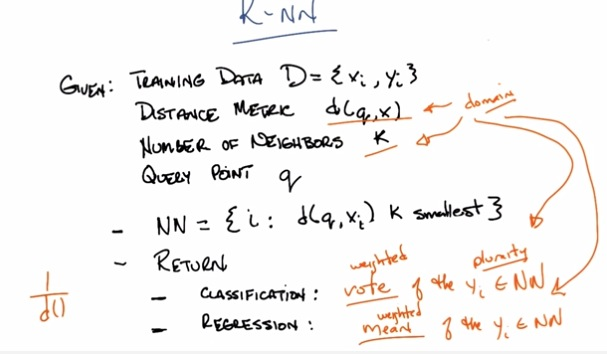• So this is the kNN algorithm that based on the housing prices example
• The Data is a pair of input and labeled data.
• there's also similarity value, that takes distance metric.
• k denotes by how many number of neighbors given some distance(similarity)
• and q, in housing example, the 2d position of the houses
• then takes to nn algorithm, as a set. calculating the smallest distance in k neighbors.
• what it will return, in terms of classification, vote, whichever have the most occurence (e.g. the house color).
• in regression, it will return mean, the average distance of all neighbors.
• and since d is reciprocal, because smalller d, the greater the value, the weight of its data towards computation.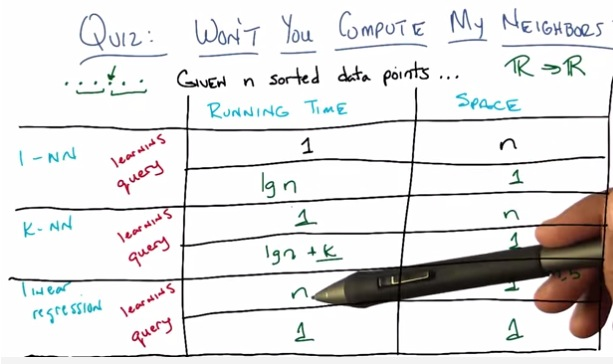• Given all the dataset, compute above each of the algorithm.
• Keep in mind that data points are sorted
• Linear Regression. The running time is n because it has to learn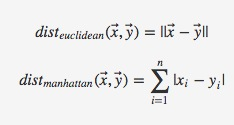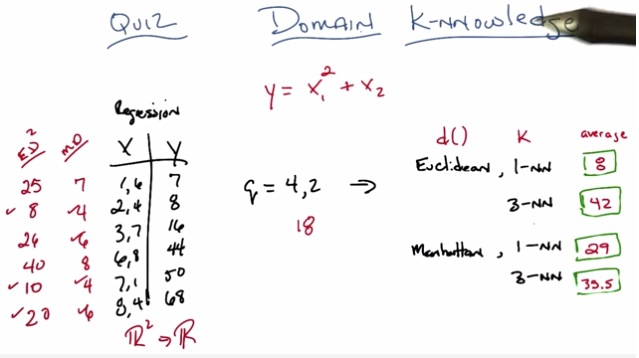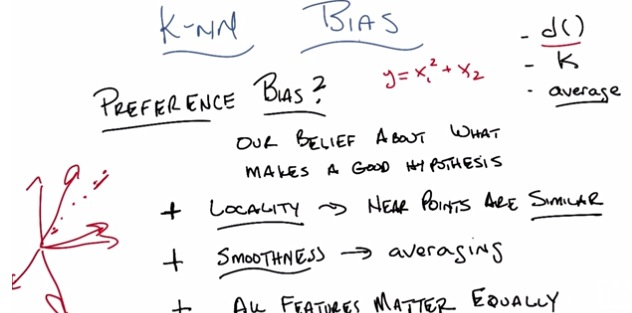• Now, kNN bias has 3 preference bias to concern.
• Locality. We must assume that every near points is similar to one another.
• Smoothness. Unlike overfitting where's high overfitting sometimes have high spike near neighbors. It assume that in 2d graph, there's model that smooth in line, have the most generalization in mind. Because of that, the kNN prefer smoothness of the model.
• in KNN, all features matter, there's no feature selection. Every features will have some impact on overall result.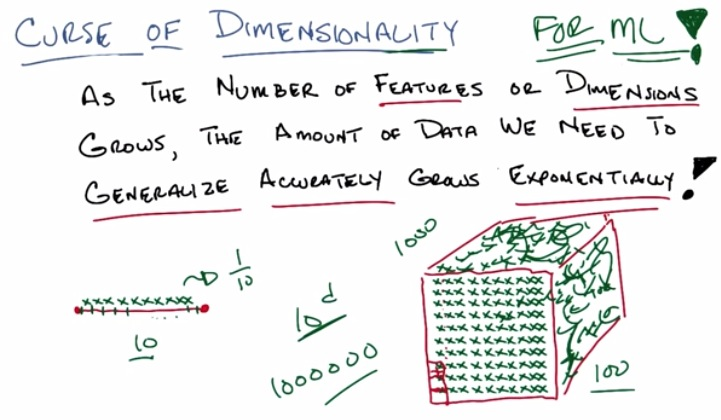• Unfortunately, in IBL, where we store all the data points, increasing a dimension will also have increase the generalization exponentially.
• This becomes harder as where we take query from it.the data that we're processing is exponentially higher according to the dimension of the data.
• As we see here if n = 10, d =1. means that each data points represent 1/10 of the space
• as d=2, space becomes 2d, each data points still have to represent 1/10 of space, so that means 10^2.
• d=3, equals 10^3, and so on.
• This makes n^d with n represent number data points, and d is the degree.
• This called what's called Curse of DImensionality, not for nn, but for all machine learning out there.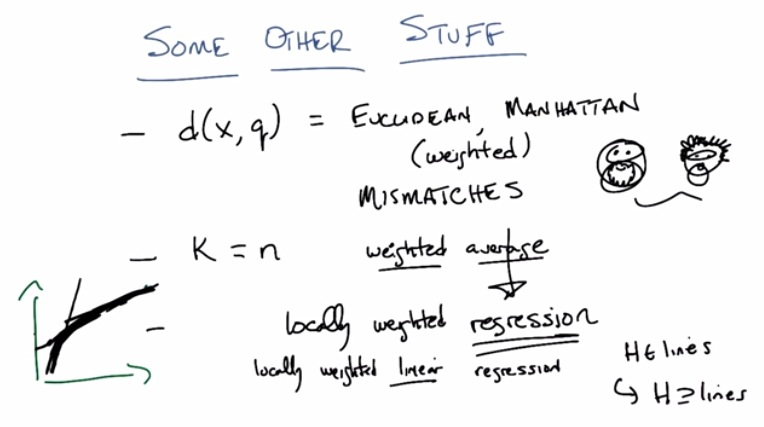• So we perform similarity, by taking distance metrix, either euclidean or manhattan
• It's important to keep in mind that the function perform the distance matters, a lot.
• not all have perfect function for distance, each have strength and weakness, sometimes we ended up mismatch the function.
• K also important. K is up to us to choose the number.
• Aside of using locally weighted average, we also can use locally weighted regression. By regression we can use decision tree, linear/logistic regression.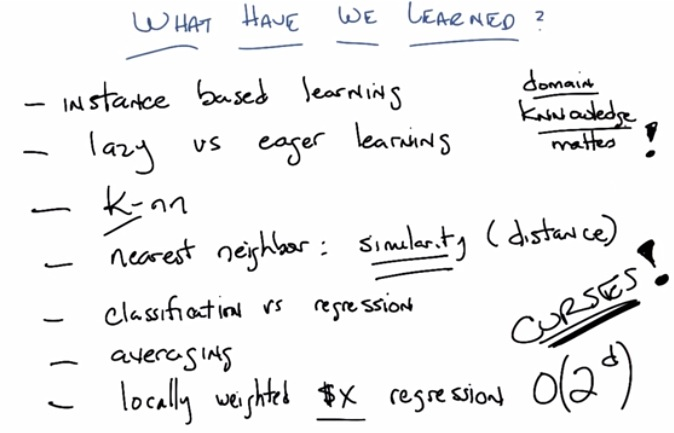• For IBL, we need apropriate function to address the distance metric(tools, knnowledge).